# Pandas常见Warning解决-SettingwithCopyWarning2020年2月24日07:04:39

1 3337字阅读11分7秒

## SettingwithCopyWarning来源分析以及解决

1. df = pd.DataFrame(np.random.randint(low=1, high=7, size=(5,4)),index=['a','b','c','d','e'],columns=['A','B','C','D'])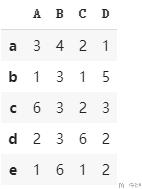### 什么是SettingwithCopyWarning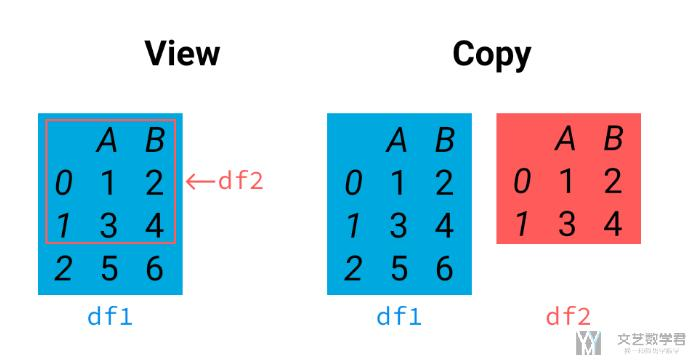A view is a way of looking at a particular portion of the original data, whereas a copy is a clone of that data to a new location in memory.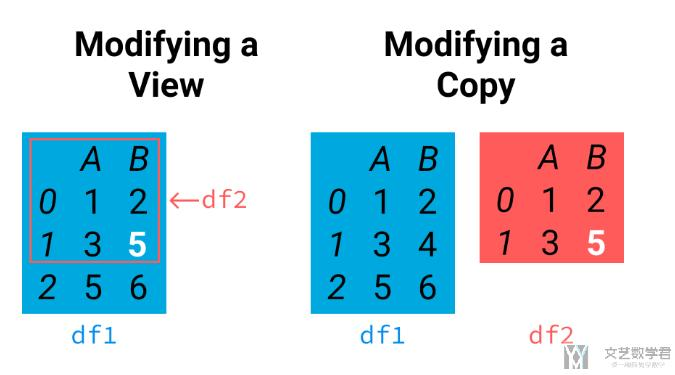### Common issue #1: Chained assignment

1. df[df.A==1]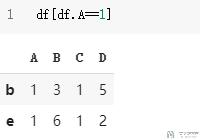1. df[df.A==1]['D']=31. # 这个时候我们重新进行查看, 可以看到值是没有改变的
2. df[df.A==1]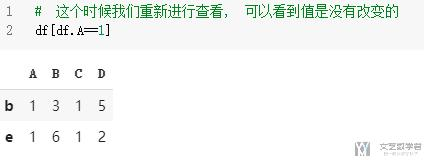The same applies to any other form of chained call because we are generating this intermediate object.

• df[df.A==1]
• ['D']=3

• 首先是第一个操作, 在进行df[df.A==1]之后, 会返回一个dataframe.
• 而第二个操作是在第一个操作生成的dataframe上进行修改的, 而不是在原始的dataframe上进行修改的, 所以最终的结果是没有改变的.

1. df.loc[df.A==1,'D']=3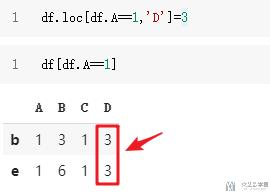### Common issue #2: Hidden chaining

1. df_sub=df[df.A==df.B]
2. df_sub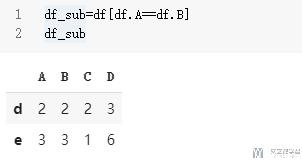1. # loc先行号, 再列号
2. df_sub.loc['d','D']=6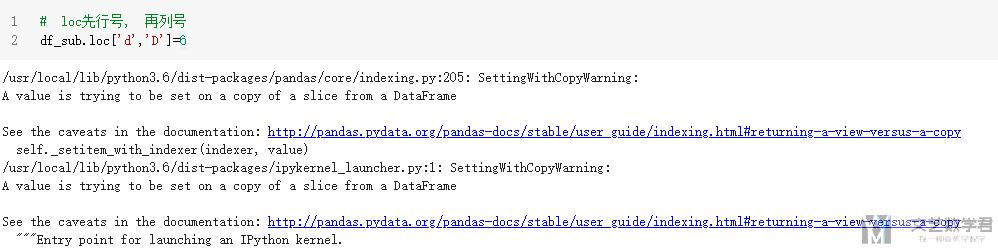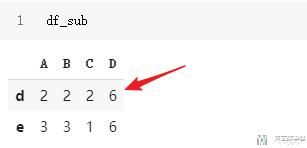1. df_sub=df[df.A==df.B].copycopy()
2. # loc先行号, 再列号
3. df_sub.loc['d','D']=6
4. df_sub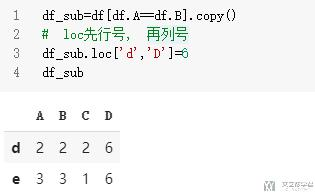### 一些更深层的原因

1. # Our code
2. data[data.bidder == 'parakeet2004']['bidderrate'] = 100

1. # Code executed
2. data.__getitem__(data.__getitem__('bidder') == 'parakeet2004').__setitem__('bidderrate', 100)

In general, as discussed, pandas does not guarantee whether a get operation will return a view or a copy of the data. If a view is returned, the second expression in our chained assignment will be a call to `setitem` on the original object.

But, if a copy is returned, it's the copy that will be modified instead – the original object does not get modified.

The `SettingWithCopyWarning` is letting us know that pandas cannot determine whether a view or a copy was returned by the first `getitem` call, and so it’s unclear whether the assignment changed the original object or not. Another way to think about why pandas gives us this warning is because the answer to the question "are we modifying the original?" is unknown.

• 微信公众号
• 关注微信公众号
•• QQ群
• 我们的QQ群号
•• 本文由 发表于 2020年2月24日07:04:39
• 转载请务必保留本文链接：https://mathpretty.com/11791.html